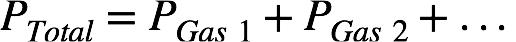# Problem: A tank contains a mixture of 52.5 g oxygen gas and 65.1 g carbon dioxide gas at 27°C. The total pressure in the tank is 9.21 atm. Calculate the partial pressures of each gas in the container.

###### FREE Expert Solution

Recall: Dalton’s Law of Partial Pressures states that the total pressure of a mixture of unreacting gases is the sum of the partial pressures of the individual gases.The partial pressure of a gas in the mixture is the product of the total pressure of the mixture and the mole fraction, χ, of the gas:We first need to calculate the mole fraction of the gases before calculating their partial pressures. The molar mass of O2 is 2(16.00 g/mol O) = 32.00 g/mol and the molar mass of CO2 is 12.01 g/mol C + 2(16.00 g/mol O) = 44.01 g/mol.

100% (436 ratings)###### Problem Details

A tank contains a mixture of 52.5 g oxygen gas and 65.1 g carbon dioxide gas at 27°C. The total pressure in the tank is 9.21 atm. Calculate the partial pressures of each gas in the container.